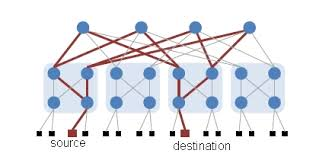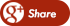﻿ How to calculate the Throughput Level in NS2?Back

## How to calculate the Throughput Level in NS2?

By Admin onTHROUGHPUT

Following awk file will calculate the throughput with running time (throughput versus time).

The following code will count all the received application packets in a network such that we can calculated the network throughput

If  a throughput of a specific node has to be calculated, then we can simply add the node_id in the if condition.

The code simply prints the observed throughput during the time_interval through out the simulation time. We can change the time_interval variable according to our requirements.

In the following code:

·      packet_size * recv * 8.0 gives the total number of bits received. Packet size is the size of packet used in Application layer diving the value by 1000 gives us the throughput in kbps.
#============== throughput.awk ================

BEGIN {
recv=0;
gotime = 1;
time = 0;
packet_size = 50;
time_interval=2;
}
#body
{
event = \$1
time = \$2
node_id = \$3
level = \$4
pktType = \$7

if(time>gotime) {

print gotime, (packet_size * recv * 8.0)/1000; #packet size * ... gives results in kbps
gotime+= time_interval;
recv=0;
}

#========Calculate throughput==========

if (( event == "r") && ( pktType == "cbr" ) && ( level=="AGT" ))
{
recv++;
}

} #body

END {
;
}And finally the throughput level has to be calculated and the above graph diagram illustrates the output of the throughput calculation.